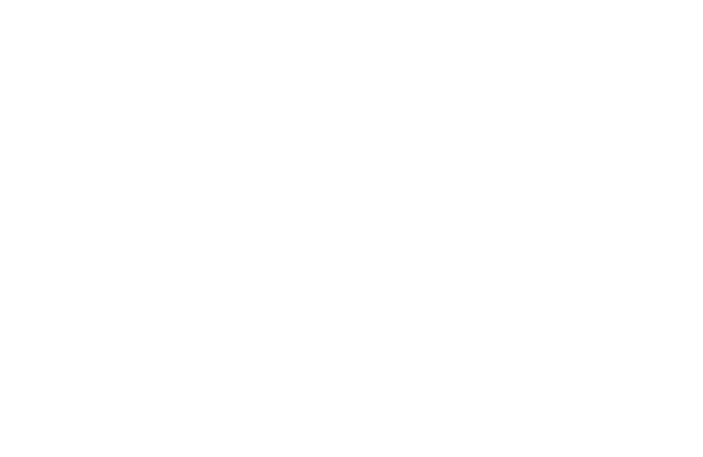🥎

# Final Code

🔗

https://codepen.io/StriveMath/full/OJWbYYr Here is the full page view you can share with the students at the start of the module

# Milestone 1: Moving Ball💡

We are still drawing with `background()` by default

``````let ballX = 100
let ballY = 100
let speedX = 1
let speedY = 1

// we are still drawing with background by default
background(0)

// Update ball position
ballY += speedY;
ballX += speedX;
// Show ball

# Milestone 2: Bouncing Ball💡

Bounce when the Edge of the ball is passes the wall Not the Center Bounce when the Center is a radius away from the wall

``````// Right - Left bounces
speedX = -speedX;
}
// Top - Bottom bounces
speedY = -speedY;
}``````

# Milestone 3: Random `position` & `speed`💡

We pick a point in the canvas randomly as the initial position of the ball Some points are not allowed These points are less than radius away from the wall``````let ballX
let ballY
let speedX
let speedY

function setup() {
createCanvas(windowWidth-100, windowHeight-100);
// Initiate ball variables
speedX = random(2, 5);
speedY = random(2, 5);
}``````

# Milestone 4: Without `background()`💡

To explain what is going on, lower frame rate# Milestone 5: `fill()` ball based on its `position`

💡

Let students do it in the way they want, then teach them and because they are not really an essence💡

We will measure the distance from the ball to canvas center and map it to Hue value Range of hue values is The range of distances is from the closest point to the center , which is the center itself, at distance zero, to the farthest allowed one``````// get coordinates of the canvas center
let centerX = width / 2;
let centerY = height / 2;
// calculate the distance from the ball to the center
let d = distance(ballX, ballY, centerX, centerY);
// calculate the maximum distance the ball is allowed ot reach
// map that distacne to a hue
let hue = map(d, 0, maxD, 0, 360);

fill(hue, 100, 100);
// Show ball

// we define this function
function distance(x1, y1, x2, y2) {
return sqrt((y2 - y1) ** 2 + (x2 - x1) ** 2);
}``````

# Milestone 6: Buffer for faster ball💡

What is `draw()` ? A function that draw a frame It's called 30 times per second by default, hence we update and draw ball 30 times each second How can we increase this rate ? 1. increase `frameRate()` which is how many times `draw()` is called in a second problem is that maximum is 60 2. Buffer it With enclosing our code by a `for()` loop, it will do what is inside many times in one `draw()` call

``````function draw(){
for(let i = 0; i < 10; i++){
//Our code goes here
}
}``````